# FlipIt - generic character flipper 1.0.0 for Maya (maya script)

A basic character flipper good for just about any rig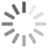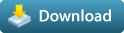#### Compatibility

• 2017, 2016, 2015, 2014, 2013, 2012, 2011, 2010, 9.x, 2009, 2008, 8.x, 7.x, 6.x, 5.x, 4.x, 3.x, 2.x

#### Operating Systems

••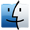•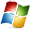#### History

 Created: 05/18/2016 Last Modified: 10/24/2017 File Size: 3.31 KB

#### Keywords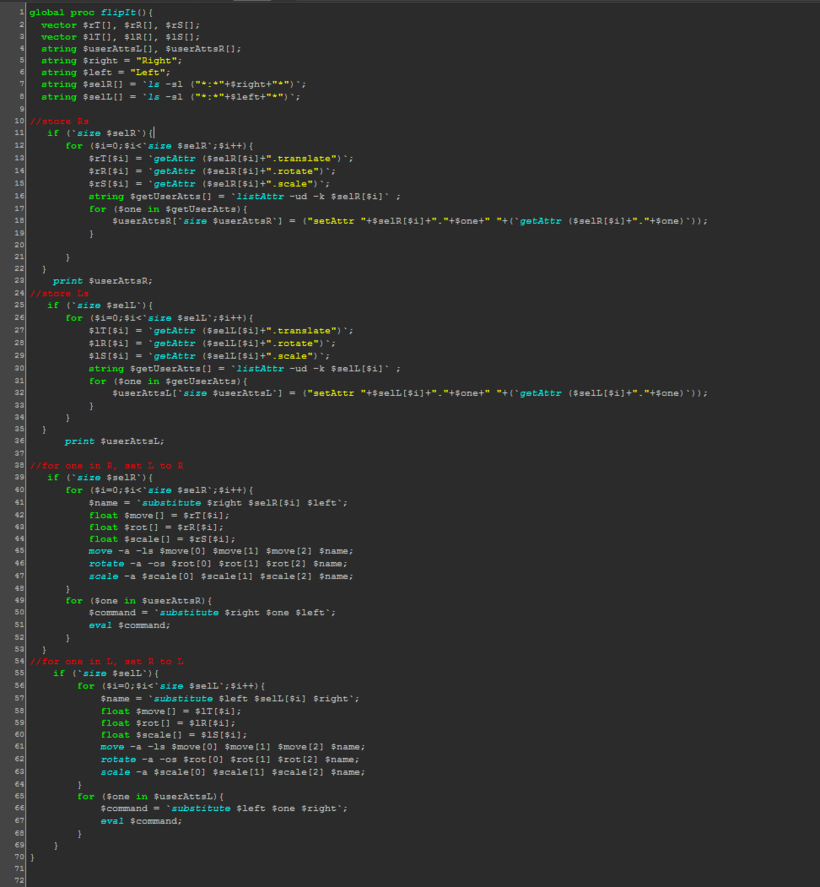Update: V 1.0 : updated the script to also incorporate user defined channels, now the flipper will work even if your naming conventions are off.

This is the bare bones script you'll need to flip a character pose from left to right. Basically, if you have just Left-hand controls selected, running this script will mirror the pose. If you have left and right side controls selected, this will Flip the pose. It works by storing the transform information of all the selected objects and then assigning them to their opposite objects.

If you're new to scripting then just know that all you have to do is change the words on line 6 and 7 to match up with the way your controllers are named. Replace the names as described, then make this script into a button. You do that by selecting the whole script and middle mouse dragging up to the shelf.

It's simple and good enough for custom rigs, or as a command button in the animSchoolPicker.

Good luck and may Odin smile upon you.

Copy the code below or download the file:

global proc flipIt(){
vector \$rT[], \$rR[], \$rS[], \$lT[], \$lR[], \$lS[];;
string \$userAttsL[], \$userAttsR[], \$right[], \$left[], \$selR[], \$selL[];;

//define naming conventions
\$right = {"right", "Right"};
\$left = {"left", "Left"};

//store user selection
for (\$one in \$right){
\$store = `ls -sl ("*:*"+\$one+"*")`;
\$size = `size \$store`;
appendStringArray(\$selR, \$store, \$size);
}

for (\$one in \$left){
\$store = `ls -sl ("*:*"+\$one+"*")`;
\$size = `size \$store`;
appendStringArray(\$selL, \$store, \$size);
}

//store R values

if (`size \$selR`){
for (\$i=0;\$i<`size \$selR`;\$i++){
\$rT[\$i] = `getAttr (\$selR[\$i]+".translate")`;
\$rR[\$i] = `getAttr (\$selR[\$i]+".rotate")`;
\$rS[\$i] = `getAttr (\$selR[\$i]+".scale")`;
string \$getUserAtts[] = `listAttr -ud -k \$selR[\$i]` ;
for (\$one in \$getUserAtts){
\$userAttsR[`size \$userAttsR`] = ("setAttr "+\$selR[\$i]+"."+\$one+" "+(`getAttr (\$selR[\$i]+"."+\$one)`));
}
}
}
print \$userAttsR;

//store L valuess

if (`size \$selL`){
for (\$i=0;\$i<`size \$selL`;\$i++){
\$lT[\$i] = `getAttr (\$selL[\$i]+".translate")`;
\$lR[\$i] = `getAttr (\$selL[\$i]+".rotate")`;
\$lS[\$i] = `getAttr (\$selL[\$i]+".scale")`;
string \$getUserAtts[] = `listAttr -ud -k \$selL[\$i]` ;
for (\$one in \$getUserAtts){
\$userAttsL[`size \$userAttsL`] = ("setAttr "+\$selL[\$i]+"."+\$one+" "+(`getAttr (\$selL[\$i]+"."+\$one)`));
}
}
}
print \$userAttsL;
doFlip(1, \$selR, \$left, \$right, \$rT, \$rR, \$rS, \$userAttsR);
doFlip(0, \$selL, \$left, \$right, \$lT, \$lR, \$lS, \$userAttsL);
}

global proc doFlip(int \$isR, string \$sel[], string \$left[], string \$right[], vector \$tr[], vector \$ro[], vector \$sc[], string \$userAtt[]){
string \$this, \$that, \$name;
if (`size \$sel`){
for (\$i=0;\$i<`size \$sel`;\$i++){
for (\$l in \$left){
for (\$r in \$right){
if (\$isR){ \$this = \$r; \$that = \$l; }
if (!\$isR){ \$this = \$l; \$that = \$r; }
if (`gmatch \$sel[\$i] ("*"+\$this+"*")`){
\$name = `substitute \$this \$sel[\$i] \$that`;
if (`objExists \$name`){
print ("from "+\$sel[\$i] + "\n");
print ("to "+ \$name + "\n");
float \$move[] = \$tr[\$i];
float \$rot[] = \$ro[\$i];
float \$scale[] = \$sc[\$i];
move -a -ls \$move \$move \$move \$name;
rotate -a -os \$rot \$rot \$rot \$name;
scale -a \$scale \$scale \$scale \$name;
for (\$one in \$userAtt){
\$command = `substitute \$this \$one \$that`;
eval \$command;
}
}
}
}
}
}
}
}

flipIt();

Please use the Bug System to report any bugs.
Please use the Feature Requests to give me ideas.
Please use the Support Forum if you have any questions or problems.
Please rate and review in the Review section.

## More Items from author BFX:

Show all 29 items from BFX